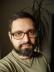Perl Weekly Challenge 044: One Hundred, Two Hundred

You are given a string “123456789”. Write a script that would insert ”+” or ”-” in between digits so that when you evaluate, the result should be 100.

We can populate each place “between digits” with one of three possible values: a plus sign, minus sign, or nothing. To check all the possible permutations, we’ll use an indicator function similarly to The Knapsack Problem. In this case, though, there are three possible values, so we need to loop over numbers in the ternary numeral system.

The only operation we’ll need will be the increment, so we don’t need the full support for arithmetic in base 3. We can implement the increment ourselves: we start from the right of the number, change any 2 into 0 and move left. Once we find 0 or 1, we increment it and we’re done.

To create the expression, we just need to intersperse the digits with the operators. See the apply subroutine below.

To evaluate the expression, we won’t use eval, as we don’t want to introduce security problems into our code. As the operations are just addition and subtraction, we can transform the expression into a large sum of positive and negative numbers (the latter correspond to the numbers being subtracted). We’ll use a regexp match to split the expression.

#!/usr/bin/perl
use warnings;
use strict;
use feature qw{ say };

use List::Util qw{ sum };

use constant {
NOTHING => 0,
PLUS    => 1,
MINUS   => 2
};

my @digits = 1 .. 9;

my %op = ( (NOTHING) => "",
(PLUS)    => '+',
(MINUS)   => '-');

sub apply {
return \$digits
. join "",
map \$op{ \$mask->[\$_-1] } . \$digits[\$_],
1 .. \$#digits
}

sub evaluate {
my (\$expression) = @_;
my @terms = \$expression =~ /[-+]?[0-9]+/g;
return sum(@terms)
}

sub increment {
}

my @mask = (NOTHING) x (@digits - 1);
while (1) {
say \$expression if 100 == evaluate(\$expression);
last unless grep \$_ != MINUS, @mask;

}

The final condition will terminate the loop while the mask contains only minuses, i.e. if the indicator function is 2222222222.

The output of the program is

123+45-67+8-9
123+4-5+67-89
123-45-67+89
123-4-5-6-7+8-9
12+3+4+5-6-7+89
12+3-4+5+67+8+9
12-3-4+5-6+7+89
1+23-4+56+7+8+9
1+23-4+5+6+78-9
1+2+34-5+67-8+9
1+2+3-4+5+6+78+9

On Linux, we can pipe the output to bc to see all the expressions evaluate to 100, as expected. There are 11 different solutions; but you may find 12 online—that’s because a variant of this task allows to put the operator at the very start of the expression, too (I don’t consider it “between digits”). It’s easy to tweak the solution to also output the twelfth solution -1+2-3+4+5+6+78+9; let’s keep it as an exercise for the reader.

Make it \$200

You have only \$1 left at the start of the week. You have been given an opportunity to make it \$200. The rule is simple with every move you can either double what you have or add another \$1. Write a script to help you get \$200 with the smallest number of moves.

Trying all the possible combinations would take ages in this case. Instead, we’ll proceed in moves and we’ll keep track of the quantities we were able to achieve so far. For each quantity, we’ll record what the shortest path to it was.

#!/usr/bin/perl
use warnings;
use strict;
use feature qw{ say };

my %possibilities = (1 => []);
while (! exists \$possibilities{200}) {
for my \$p (keys %possibilities) {
\$possibilities{ \$_ } ||= [ @{ \$possibilities{\$p} }, \$p ]
for \$p + 1, \$p * 2;
}
}

my @moves = @{ \$possibilities{200} };
say scalar @moves, ": @moves";

As you can see, in each move we try to extend each number obtained so far in both the possible ways, and if the result is a new number, we record it in the hash.I blog about Perl.